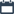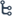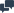## Wednesday, February 26, 2020

•February 26, 2020
••, , ,
•No comments

## Simplify the ratio 20 : 5 & 500 g to 250 g.

1.Simplify the ratio 20 : 5
Solution

Step 1 : Write the ratio in fraction form as 5 20 .
Step 2 : Divide each quantity by 5. That is, 20 ÷ 5 = 4
5 ÷ 5     1
= 4 : 1
Answer : the ratio in the simplest form 4 : 1

2. Find the ratio of 500 g to 250 g.
Solution

500 g to 250 g = 500 : 250
500   = 500  ÷ 250  = 2  = 2 : 1
250      250 ÷250      1

Answer :  the ratio in the simplest form of 2 : 1

## Categories